# Generalized Linear Models¶

Examples concerning the sklearn.linear_model module.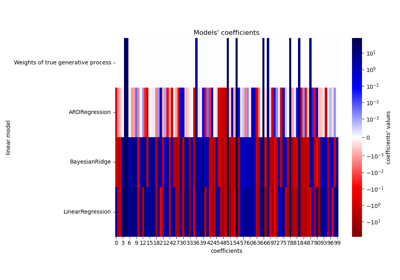Comparing Linear Bayesian Regressors

Comparing Linear Bayesian RegressorsComparing various online solvers

Comparing various online solvers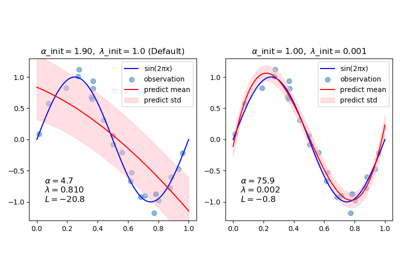Curve Fitting with Bayesian Ridge Regression

Curve Fitting with Bayesian Ridge Regression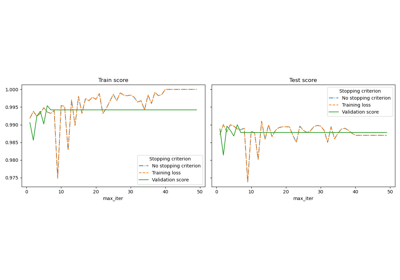Early stopping of Stochastic Gradient Descent

Early stopping of Stochastic Gradient DescentFitting an Elastic Net with a precomputed Gram Matrix and Weighted Samples

Fitting an Elastic Net with a precomputed Gram Matrix and Weighted Samples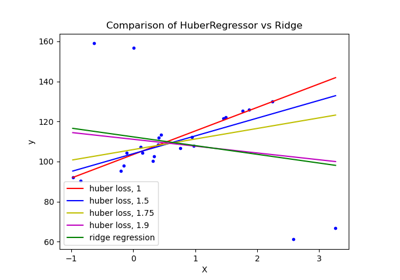HuberRegressor vs Ridge on dataset with strong outliers

HuberRegressor vs Ridge on dataset with strong outliers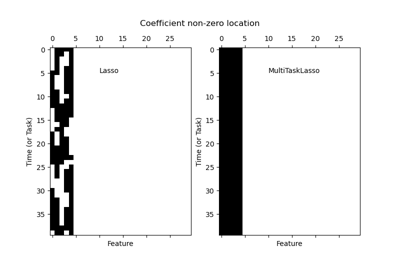Joint feature selection with multi-task Lasso

Joint feature selection with multi-task Lasso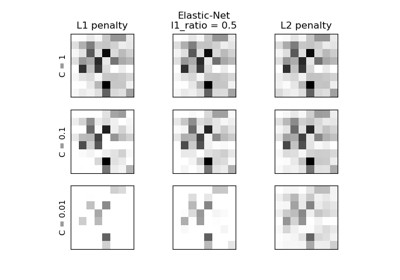L1 Penalty and Sparsity in Logistic Regression

L1 Penalty and Sparsity in Logistic Regression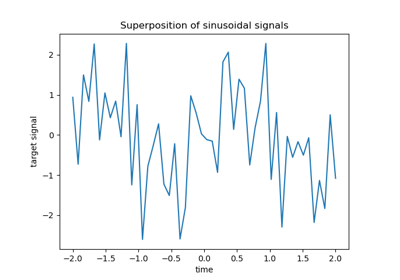L1-based models for Sparse Signals

L1-based models for Sparse SignalsLasso and Elastic Net

Lasso and Elastic Net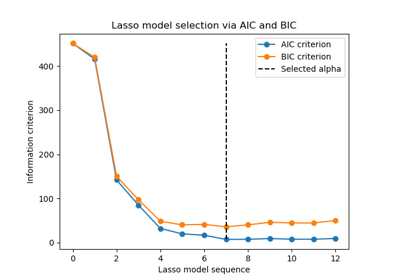Lasso model selection via information criteria

Lasso model selection via information criteria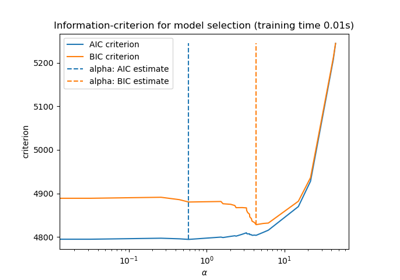Lasso model selection: AIC-BIC / cross-validation

Lasso model selection: AIC-BIC / cross-validationLasso on dense and sparse data

Lasso on dense and sparse dataLasso path using LARS

Lasso path using LARSLinear Regression Example

Linear Regression ExampleLogistic Regression 3-class Classifier

Logistic Regression 3-class ClassifierLogistic function

Logistic function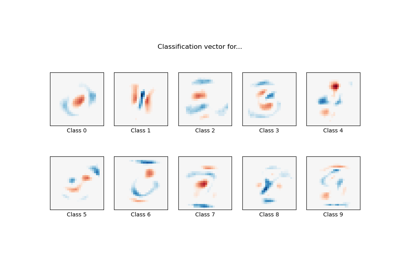MNIST classification using multinomial logistic + L1

MNIST classification using multinomial logistic + L1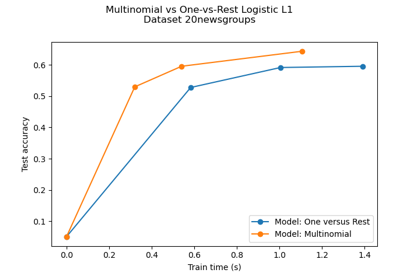Multiclass sparse logistic regression on 20newgroups

Multiclass sparse logistic regression on 20newgroups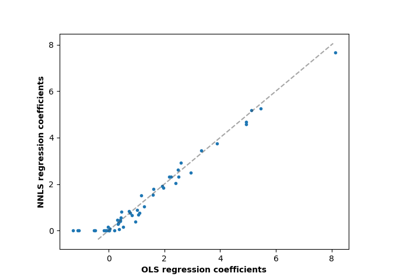Non-negative least squares

Non-negative least squares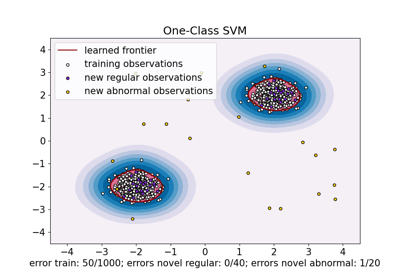One-Class SVM versus One-Class SVM using Stochastic Gradient Descent

One-Class SVM versus One-Class SVM using Stochastic Gradient DescentOrdinary Least Squares and Ridge Regression Variance

Ordinary Least Squares and Ridge Regression Variance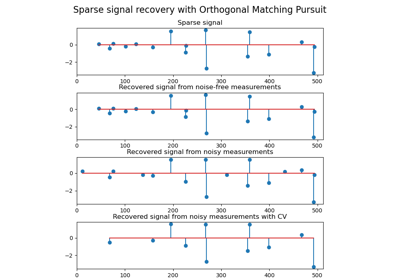Orthogonal Matching Pursuit

Orthogonal Matching Pursuit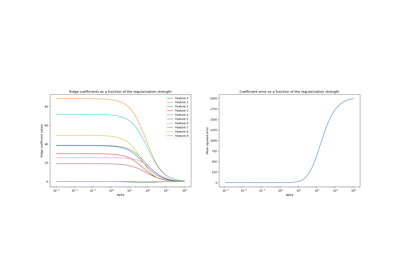Plot Ridge coefficients as a function of the L2 regularization

Plot Ridge coefficients as a function of the L2 regularization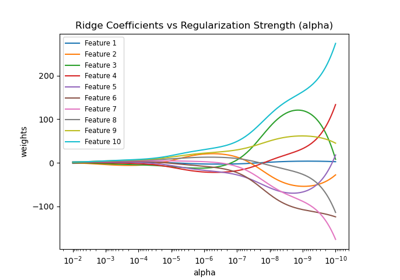Plot Ridge coefficients as a function of the regularization

Plot Ridge coefficients as a function of the regularization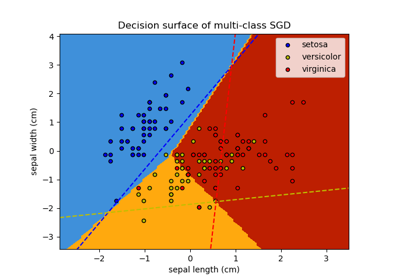Plot multi-class SGD on the iris dataset

Plot multi-class SGD on the iris dataset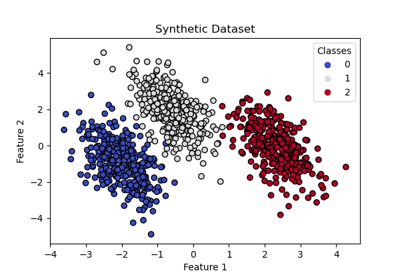Plot multinomial and One-vs-Rest Logistic Regression

Plot multinomial and One-vs-Rest Logistic Regression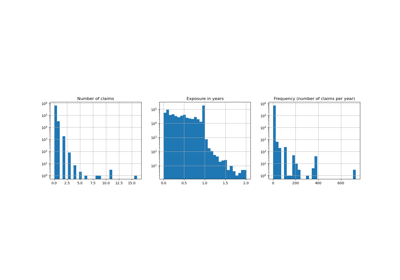Poisson regression and non-normal loss

Poisson regression and non-normal loss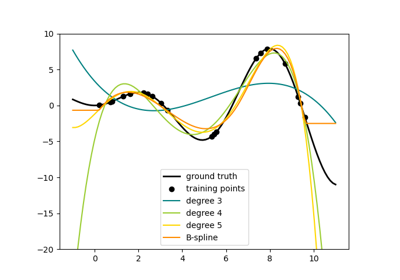Polynomial and Spline interpolation

Polynomial and Spline interpolation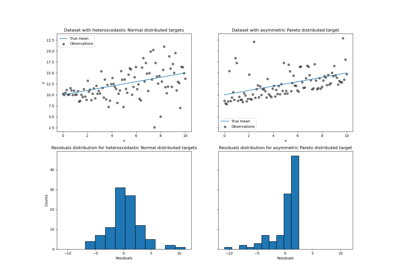Quantile regression

Quantile regression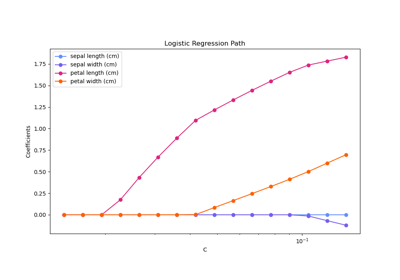Regularization path of L1- Logistic Regression

Regularization path of L1- Logistic Regression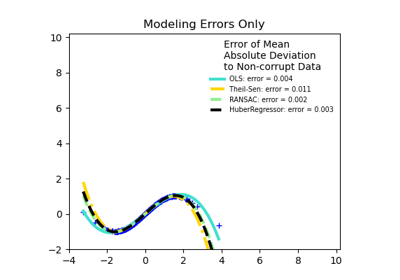Robust linear estimator fitting

Robust linear estimator fitting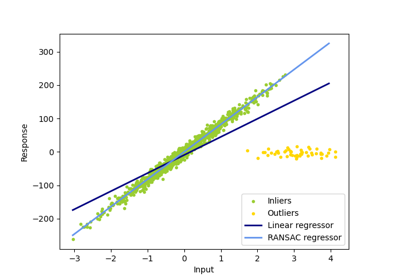Robust linear model estimation using RANSAC

Robust linear model estimation using RANSAC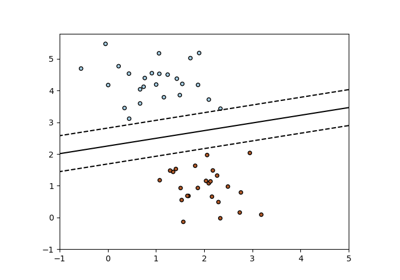SGD: Maximum margin separating hyperplane

SGD: Maximum margin separating hyperplane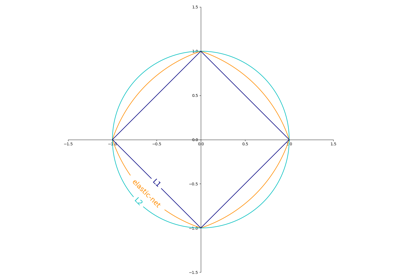SGD: Penalties

SGD: Penalties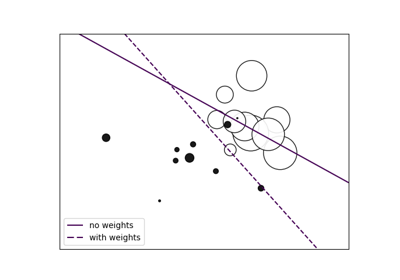SGD: Weighted samples

SGD: Weighted samples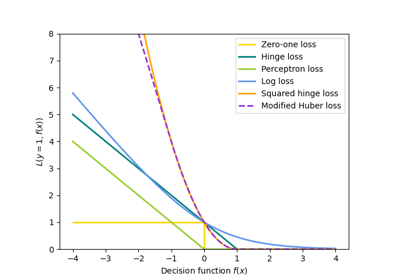SGD: convex loss functions

SGD: convex loss functionsSparsity Example: Fitting only features 1 and 2

Sparsity Example: Fitting only features 1 and 2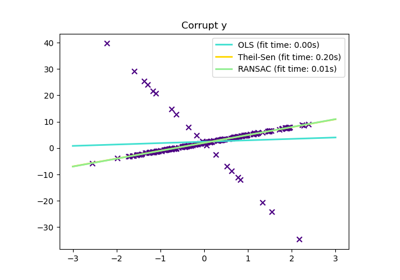Theil-Sen Regression

Theil-Sen Regression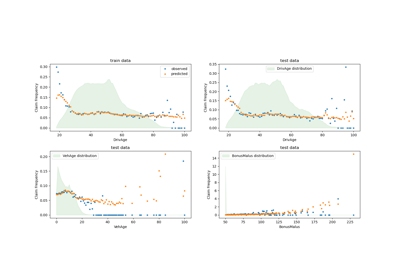Tweedie regression on insurance claims

Tweedie regression on insurance claims